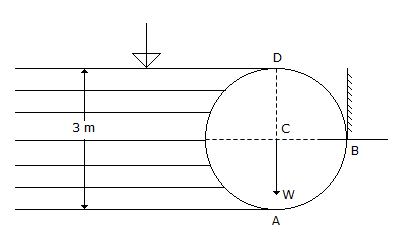# Civil Engineering - Hydraulics - Discussion

Discussion Forum : Hydraulics - Section 2 (Q.No. 46)
46.
A cylinder 3 m in diameter and 4 m long retains water one side as shown in the below figure. If the weight of the cylinder is 2000 kgf, the vertical reaction at A is14,137 kgf
5,863 kgf
20,000 kgf
18,000 kgf.
Explanation:
No answer description is available. Let's discuss.
Discussion:
13 comments Page 1 of 2.

JENISH POUDEL said:   1 month ago
Reaction = vertical force by water on A+2000kg
Vertical force = wt of water in a volume[volume here is vertically upward projected portion of water without the semicylindrical portion]
Vertical force = 1000 * vol = 1000 * [3 * 1.4 * 4 - 0.5 * 3.14 * 3^(2) * 4/4 = 1000 * [18 - 14.137].
= 3863kg force.
Then the reaction = 3863 + 2000 = 5863kg.

Rakesh roushan said:   2 years ago
Thank you for explaining @Nushi.

Aadil bhat said:   3 years ago
@Nungshi Jr.

Yes, you are right. Thanks.

Nushi said:   5 years ago
Vertical reaction = wt Of cylinder - vertical Force.
Vertical force= specific weight * volume,
= 1000 * &pie; *1.5^2 * 4/2.
= 14137 kgf.

Ra= 20000-14137 = 5863 kgf.
(2)

Nungshi Jr said:   5 years ago
Reaction at B = horizontal force (Fh).
Fh= specific wt * area * y.
= 1000 * 3 * 4 * 3/2,
= 18000 kgf.

Basavaraj said:   5 years ago
F(verticle) = 3.142 * R^2 * L * 9.81.

Kumar Adarsh said:   5 years ago
Yes, right @Baleno.

Anshu said:   5 years ago